A# trill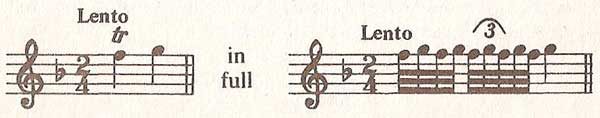Figure 1. Examples of trills.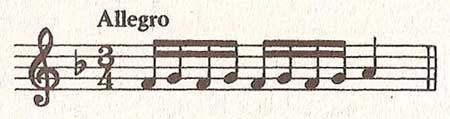Figure 3. Trill beginning on principal note followed by a note a third higher.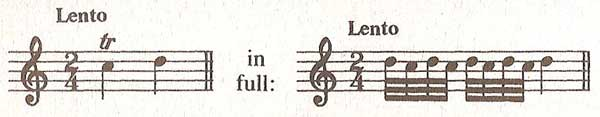Figure 4. Trill beginning on the note above the principal note.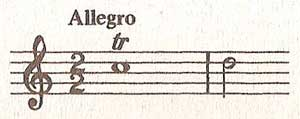Figure 5. Trill as triplets.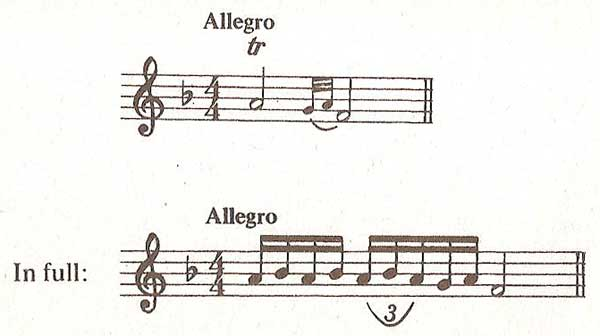Figure 6. Trill ending with turn.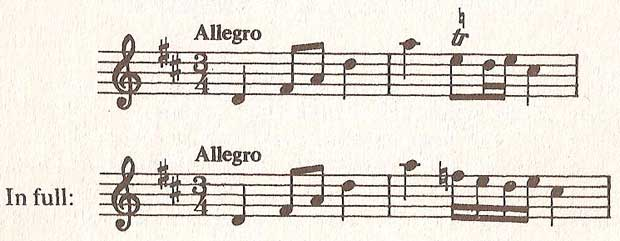Figure 7. Trill with accidental.

A trill, also known as a shake, is an ornament of two notes – a principal note and the note above. These are played quickly, one after the other, and are indicated by the first two letters of the word (tr) written over the principal note. Some examples of trills are shown in Figure 1.

The amount of alterations put into a trill depends upon the value of the principal note and the tempo of the music. For tempo less than allegro, use demisemiquavers. For allegro or faster, use semiquavers.

A trill should always end on the principal note (see Figure 2) unless followed by a note a third higher.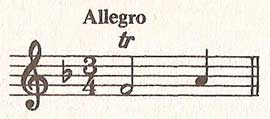Figure 2. Trill beginning on principal note.

In this case, omit the last repetition of the principal note (see Figure 3).

In the music of Mozart's time the trill was begun on the note above the principal note (see Figure 4).

This produces an even number of notes, ending on the principal note.

In later music the trill is intended to begin on the principal note. This results in an uneven number of notes in the last group and, therefore, the writing of a triplet.

To make a musical rendering, the triplet falls on the first, second, and third of the last five notes (see Figure 5).

Of course, if the principal note is immediately preceded by a note of the same pitch it would not be musical to begin a trill on that note, but on the note above, as in earlier times.

If the trill is immediately preceded by an acciaccatura, that note must be considered to be the first note of the trill.

If the principal note is prolonged, either by a dot or a tie, the trill should continue for the total value.

If a composer wants a trill to end with a turn, they will write the principal note either with its full value and indicate the turn by two tiny notes which may be of any value, but usually a semiquaver or demisemiquaver; or they will show the last two notes as normal sized notes and detract their value from the principal note (see Figure 6).

If a turn is added at the end of a trill, the notes are of the same value and are grouped in the same way as the basic trill.

Unless a turn is indicated in either way above, no turn should be introduced.

Normally a trill, with or without a turn added at the end, uses notes diatonic to the key of the music. Sometimes, however, to change key (modulate) a composer alters the upper note of the trill by placing an accidental above the sign (see Figure 7).

Notice that, in the example above, the trill starts on the upper note (the normal practise) and, because of the speed, uses semiquavers and is only four notes – sounding like a four-note turn. This example has enabled the composer to modulate from D major to C major.

In vocal terminology, trillo (Italian), originally referred to a trill, but in recent pedagogy means a rapid repetition of the same note, which usually includes repeated voice onset and offset.